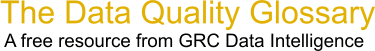Choose Index below for a list of all words and phrases defined in this glossary.

# Douglass Index

index | Index

## Douglass Index - definition(s)

Douglass Index - Formula used to improve a capability Cp by subtracting out a marginal RR rate to factor and segregate equipment variation. Example: The process capability is the reciprocal of the Cp x 100%. This is the summation of the sum of the squares of the contributing factors (i.e.) Capability squared x RR squared. The reciprocal of a marginal cPk of 1.50 is .666 x 100% = 66.6% machine capability. The square of that is 4435. The square of a poor RR of 29 is 841. Subtracting 841 from 4435 is 3594. The square root of 3594 is 59 divided by 100 is .599. The reciprocal of 0.599 is 1.67 (this is the improved cP 1.67).

[Category=Data Quality ]

Source: Jim Douglass, quoted on iSixSigma, 18 January 2011 10:33:37, https:web.archive.org/web/20111109014246/http:www.isixsigma.com/index.php?option=com_glossaryData Quality Glossary.  A free resource from GRC Data Intelligence. For comments, questions or feedback: dqglossary@grcdi.nl